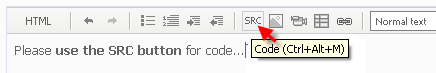# fix my script --> open pending order11

i'm a newbie at mql4 coding, please help me to fix my script or make a new one.

i have a script, that will set a pending order by the time setting.

I have 2 pending orders each time 1 BUY, 1 SELL.

If 1 of these trades is triggered and hits its take profit, I want to cancel the other order or close when the orther was trigered

If Hour2 is reached and one or both of the previous pending orders have not opened, they are deleted

extern double lot = 0.1;
extern int step = 10;
extern int tp = 5;
extern int magic = 1;
extern int slip = 3;
extern int jamasia = 0;
extern int menitasia = 1;
extern int jameropa = 7;
extern int meniteropa = 1;
extern int jamamerika = 12;
extern int menitamerika = 1;

bool open;
int ticket;
//+------------------------------------------------------------------+
//| expert initialization function |
//+------------------------------------------------------------------+
int init()
{
//----

//----
return(0);
}
//+------------------------------------------------------------------+
//| expert deinitialization function |
//+------------------------------------------------------------------+
int deinit()
{
//----

//----
return(0);
}
//+------------------------------------------------------------------+
//| expert start function |
//+------------------------------------------------------------------+
int start()
{
//----
if((Hour()== jamasia && Minute() == menitasia) && OrdersTotal() == 0){
sellstop(lot,step,slip,tp,magic);
}
else if((Hour()== jameropa && Minute() == meniteropa) && OrdersTotal() == 0){
sellstop(lot,step,slip,tp,magic);

}
else if((Hour()== jamamerika && Minute() == menitamerika) && OrdersTotal() == 0){
sellstop(lot,step,slip,tp,magic);

}
if(OrdersTotal() > 0 && totalbuy() > 0 || totalsell() > 0){
closeall();
}

//----
return(0);
}
//+------------------------------------------------------------------+

//---
{
int totalb=0;
int tipe;
for(int i=0; i<OrdersTotal(); i++)
{
if(OrderSymbol()!=Symbol()) continue;
tipe=OrderType();
}
return(totalb);
}

int totalsell()
{
int totals=0;
int tipe;
for(int i=0; i<OrdersTotal(); i++)
{
if(OrderSymbol()!=Symbol()) continue;
tipe=OrderType();
if(tipe==OP_SELL) totals++;
}
return(totals);
}

//--------penghapus order------------
void closeall()
{ for (int i = OrdersTotal()-1 ; i >= 0; i--)
if (OrderSymbol() == Symbol() && OrderMagicNumber() == 1||2 )//&& OrderCloseTime() == 0)
{ if(OrderType() == OP_SELLLIMIT) OrderDelete(OrderTicket());
if(OrderType() == OP_SELLSTOP) OrderDelete(OrderTicket());
if(OrderType() == OP_BUYSTOP>0 && OP_SELLSTOP == 0 && totalsell() == 0){
closeall();
}
else if(OrderType() == OP_SELLSTOP > 0 && OP_BUYSTOP == 0 && totalbuy() == 0){
closeall();
}
Sleep(2000);
}
}
}

void buystop(double lot,int step,int slip,int tp,int magic){
open = true;
while( open == true)
{ ticket = OrderSend( Symbol(), OP_BUYSTOP, lot, Ask + step*Point, slip, 0, Ask + (tp+step)*Point, NULL, magic, 0, CLR_NONE);
if( ticket < 0 ) open = true;
else open = false;
RefreshRates();
}
}

//-------OP sell-----
void sellstop(double lot,int step,int slip,int tp,int magic){
open = true;
while( open == true)
{ ticket = OrderSend( Symbol(), OP_SELLSTOP, lot, Bid - step*Point, slip, 0, Bid - (tp+step)*Point, NULL, magic, 0, CLR_NONE);
if( ticket < 0 ) open = true;
else open = false;
RefreshRates();
}

}53

First suggestion, use comments. Code becomes very overwhelming when you never separate sections and document their use (or implied use).

Secondly, from what I can see, you don't have a mechanism for triggering any kind of hour tracking. So maybe if you could refine your question and we could tackle one aspect at a time that would be more productive in solving them.11

thekaptain:

First suggestion, use comments. Code becomes very overwhelming when you never separate sections and document their use (or implied use).

Secondly, from what I can see, you don't have a mechanism for triggering any kind of hour tracking. So maybe if you could refine your question and we could tackle one aspect at a time that would be more productive in solving them.

thanks for comment, ok let make it simple. no need to watch the time order

my problem just how to correct my delete pending order.

void closeall()
{ for (int i = OrdersTotal()-1 ; i >= 0; i--)
if (OrderSymbol() == Symbol() && OrderMagicNumber() == 1||2 )//&& OrderCloseTime() == 0)
{ if(OrderType() == OP_SELLLIMIT) OrderDelete(OrderTicket());
if(OrderType() == OP_SELLSTOP) OrderDelete(OrderTicket());
if(OrderType() == OP_BUYSTOP>0 && OP_SELLSTOP == 0 && totalsell() == 0){
closeall();
}
else if(OrderType() == OP_SELLSTOP > 0 && OP_BUYSTOP == 0 && totalbuy() == 0){
closeall();
}
Sleep(2000);
}
}
}

modify mw older script with this rule

suppose there are two pending order by the time set before. BUYSTOP and SELLSTOP

If one of the orders opens, the other remains pending

If the open order reaches TakeProfit, the other pending order is deleted (case when the others still pending/the order not opens)

If the open order reaches Take Profit, the other open orders are close (cutlose) --> case when two orders are opened

thank you11

If one of the orders opens, the other remains pending

If the open order reaches TakeProfit, the other pending order is deleted (case when the others still pending/the order not opens)

If the open order reaches Take Profit, the other open orders are close (cutlose) --> case when two orders are opened

Files:53

First off, you can determine if an order was opened by OrderOpenTime()

ex OrderSelect(...){

if(OrderOpenTime() != 0){

//Order was opened, close the other order

}

}

But that is just the beginning, because you also want to know if an order reached Take Profit. In order to do that, you must call an OrderSelect(...,..., MODE_HISTORY);. Because MODE_TRADES is only for open orders.

So, you must call another function (orderselect with mode_history) and filter your exit according to either the OrderClosePrice() in comparison to the Take Profit or you could compare it to the OrderProfit() and then close the opposite order.17271

andateruna:

i'm a newbie at mql4 coding, please help me to fix my script or make a new one.

Before posting please read some of the other threads . . . then you would have seen numerous requests like this one:

Please use this to post code . . . it makes it easier to read.